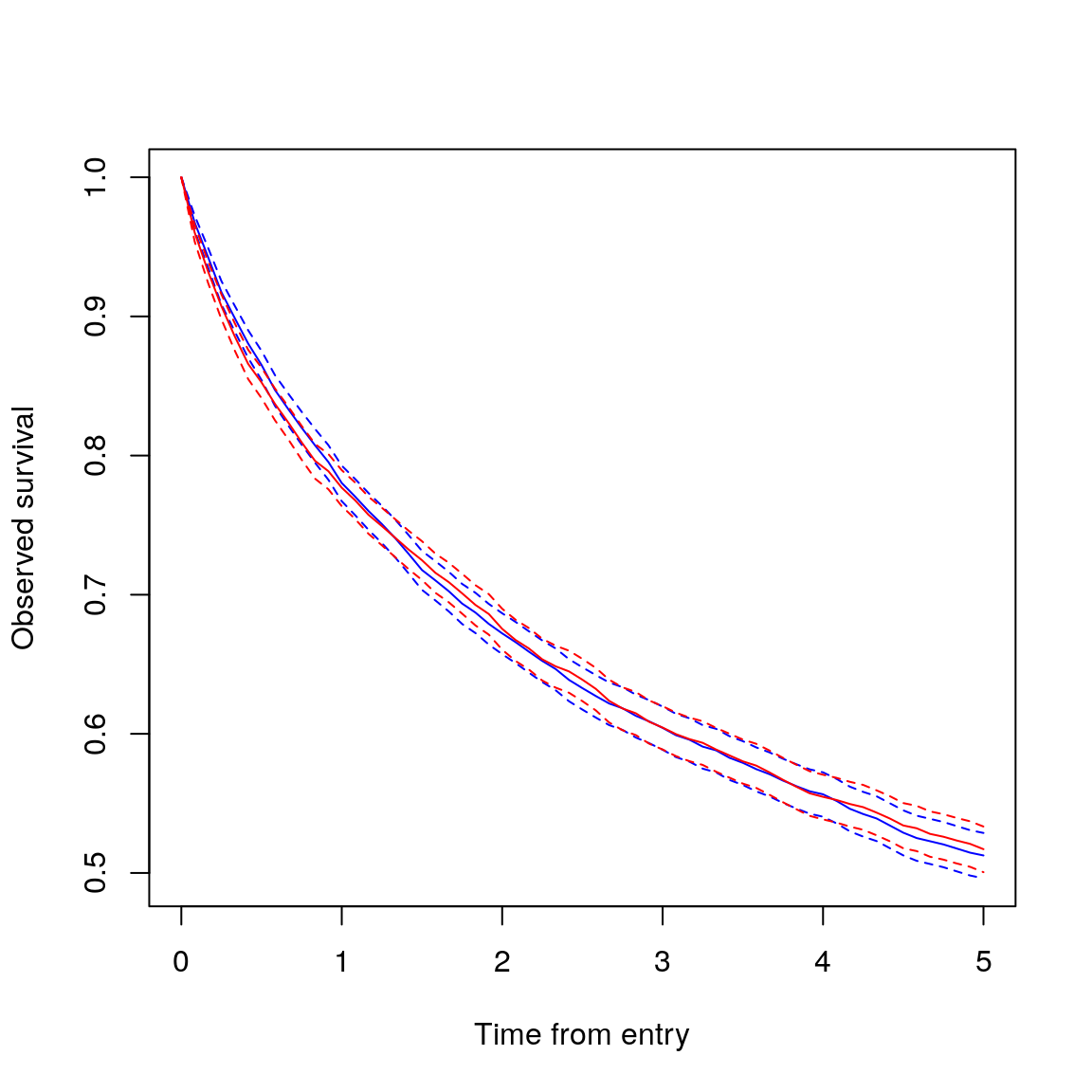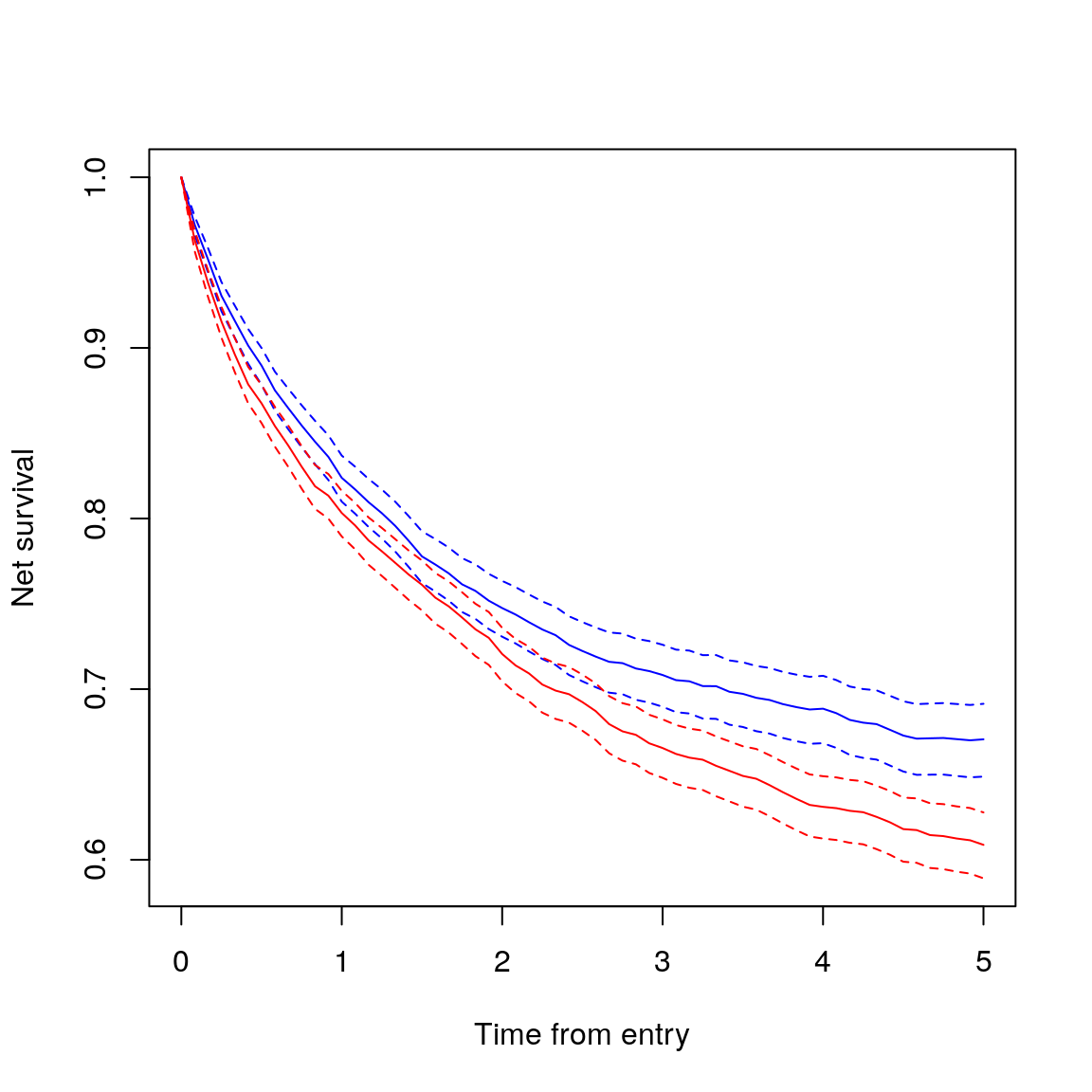# Overview

This vignette aims to clarify the usage of the `survtab_ag` and `survtab` functions included in this package. `survtab_ag` estimates various survival functions and cumulative incidence functions (CIFs) non-parametrically using aggregated data, and `survtab` is a wrapper for `survtab_ag`, to which `Lexis` data is supplied.

Two methods (`surv.method`) are currently supported: The `"lifetable"` (actuarial) method only makes use of counts when estimating any of the supported survival time functions. The default method (`"hazard"`}) estimates appropriate hazards and transforms them into survival function or CIF estimates.

For relative survival estimation we need also to enumerate the expected hazard levels for the subjects in the data. This is done by merging expected hazards to individuals’ subintervals (which divide their survival time lines to a number of small intervals). For Pohar-Perme-weighted analyses one must additionally compute various weighted figures at the level of split subject data.

If one has subject-level data, the simplest way of computing survival function estimates with `popEpi` is by defining a `Lexis` object and using `survtab`, which will do the rest. For pre-aggregated data one may use the `survtab_ag` function instead. One can also use the `lexpand` function to split, merge population hazards, and aggregate in a single function call and then use `survtab_ag` if that is convenient.

# Using `survtab`

It is straightforward to estimate various survival time functions with `survtab` once a `Lexis` object has been defined (see `?Lexis` in package `Epi` for details):

``````library(popEpi)
library(Epi)
library(survival)``````
``````data(sire)

## NOTE: recommended to use factor status variable
x <- Lexis(entry = list(FUT = 0, AGE = dg_age, CAL = get.yrs(dg_date)),
exit = list(CAL = get.yrs(ex_date)),
data = sire[sire\$dg_date < sire\$ex_date, ],
exit.status = factor(status, levels = 0:2,
labels = c("alive", "canD", "othD")),
merge = TRUE)``````
``## NOTE: entry.status has been set to "alive" for all.``
``````## pretend some are male
set.seed(1L)
x\$sex <- rbinom(nrow(x), 1, 0.5)

## observed survival - explicit method
st <- survtab(Surv(time = FUT, event = lex.Xst) ~ sex, data = x,
surv.type = "surv.obs",
breaks = list(FUT = seq(0, 5, 1/12)))

## observed survival - easy method (assumes lex.Xst in x is the status variable)
st <- survtab(FUT ~ sex, data = x,
surv.type = "surv.obs",
breaks = list(FUT = seq(0, 5, 1/12)))

## printing gives the used settings and
## estimates at the middle and end of the estimated
st``````
``````##
## Call:
##  survtab(formula = FUT ~ sex, data = x, breaks = list(FUT = seq(0, 5, 1/12)), surv.type = "surv.obs")
##
## Type arguments:
##  surv.type: surv.obs --- surv.method: hazard
##
## Confidence interval arguments:
##  level: 95 % --- transformation: log-log
##
## Totals:
##  person-time:23993 --- events: 3636
##
## Stratified by: 'sex'
##    sex Tstop surv.obs.lo surv.obs surv.obs.hi SE.surv.obs
## 1:   0   2.5      0.6174   0.6328      0.6478    0.007751
## 2:   0   5.0      0.4962   0.5126      0.5288    0.008321
## 3:   1   2.5      0.6235   0.6389      0.6539    0.007748
## 4:   1   5.0      0.5006   0.5171      0.5334    0.008370``````

Plotting by strata (men = blue, women = red):

``plot(st, col = c("blue", "red"))``Note that the correct usage of the `formula` argument in `survtab` specifies the time scale in the `Lexis` object over which survival is computed (here `"FUT"` for follow-up time). This is used to identify the appropriate time scale in the data. When only supplying the survival time scale as the right-hand-side of the formula, the column `lex.Xst` in the supplied `Lexis` object is assumed to be the (correctly formatted!) status variable. When using `Surv()` to be explicit, we effectively (and exceptionally) pass the starting times to the `time` argument in `Surv()`, and `time2` is ignored entirely. The function will fail if `time` does not match exactly with a time scale in data.

When using `Surv()`, one must also pass the status variable, which can be something other than the `lex.Xst` variable created by `Lexis()`, though usually ``lex.Xst` is what you want to use (especially if the data has already been split using e.g. `splitLexis` or `splitMulti`, which is allowed). It is recommended to use a factor status variable to pass to `Surv()`, though a numeric variable will work in simple cases (0 = alive, 1 = dead; also `FALSE` = alive, `TRUE` = dead). Using `Surv()` also allows easy passing of transformations of `lex.Xst`, e.g. `Surv(FUT, lex.Xst %in% 1:2)`.

The argument `breaks` must be a named list of breaks by which to split the `Lexis` data (see `?splitMulti`). It is mandatory to assign breaks at least to the survival time scale (`"FUT"` in our example) so that `survtab` knows what intervals to use to estimate the requested survival time function(s). The breaks also determine the window used: It is therefore easy to compute so called period estimates by defining the roof and floor along the calendar time scale, e.g.

`breaks = list(FUT = seq(0, 5, 1/12), CAL = c(2000, 2005))`

would cause `survtab` to compute period estimates for 2000-2004 (breaks given here as fractional years, so 2005 is effectively 2004.99999…).

## Relative/net survival

Relative/net survival estimation requires knowledge of the expected hazard levels for the individuals in the data. In `survtab` this is accomplished by passing a long-format `data.frame` of population hazards via the `pophaz` argument. E.g. the `popmort` dataset included in `popEpi` (Finnish overall mortality rates for men and women).

``````data(popmort)
pm <- data.frame(popmort)
names(pm) <- c("sex", "CAL", "AGE", "haz")
``````##   sex  CAL AGE         haz
## 1   0 1951   0 0.036363176
## 2   0 1951   1 0.003616547
## 3   0 1951   2 0.002172384
## 4   0 1951   3 0.001581249
## 5   0 1951   4 0.001180690
## 6   0 1951   5 0.001070595``````

The `data.frame` should contain a variable named `"haz"` indicating the population hazard at the level of one subject-year. Any other variables are considered to be variables, by which to merge population hazards to the (split) subject-level data within `survtab`. These merging variables may correspond to the time scales in the used `Lexis` object. This allows for e.g. merging in different population hazards for the same subject as they get older.

The following causes `survtab` to estimate EdererII relative survival:

``````st.e2 <- survtab(Surv(time = FUT, event = lex.Xst) ~ sex, data = x,
surv.type = "surv.rel", relsurv.method = "e2",
breaks = list(FUT = seq(0, 5, 1/12)),
pophaz = pm)``````
``plot(st.e2, y = "r.e2", col = c("blue", "red"))``Note that the curves diverge due to merging in the “wrong” population hazards for some individuals which we randomized earlier to be male though all the individuals in data are actually female. Pohar-Perme-weighted estimates can be computed by

``````st.pp <- survtab(Surv(time = FUT, event = lex.Xst) ~ sex, data = x,
surv.type = "surv.rel", relsurv.method = "pp",
breaks = list(FUT = seq(0, 5, 1/12)),
pophaz = pm)``````

Compare with EdererII estimates:

``````plot(st.e2, y = "r.e2", col = c("blue", "red"), lty = 1)
lines(st.pp, y = "r.pp", col = c("blue", "red"), lty = 2)``````